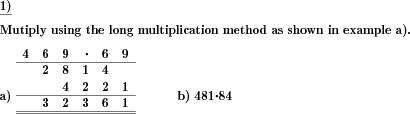Custom math worksheets at your fingertips# Details for problem "Long multiplication to be performed for two factors"

Quickname: 3232

Elementary School, Primary School, Middle School.

## Summary

Two factors have to be multiplied using the long multiplication written form.

## Example## Description

Two whole positive natural numbers have to be multiplied using the long multiplication written notation.

The number of places can be configured individually for the first and second factor. Optionally, a simplification may be introduced that will always make the second factor a multiple of ten.

To make is easier to follow the long addition operation, carries may be included in the visualization of the answer. The first problem can be configured to be a sample problem that will include the solution.

The number of problems is selectable.

Download free printable worksheets for this math problem here. The worksheet contains the problems only, the solution sheet includes the answers. Just click on the respective link.

•Worksheet 1Solution sheet with answers
•Worksheet 2Solution sheet with answers
•Worksheet 3Solution sheet with answers

If you can not see the solution sheets for download, they may be filtered out by an ad blocker that you may have installed. If this is the case, please allow ads for this page and reload the page. The solution sheets will then reappear.

• Do these sample worksheets do not really fit?
• Do you need more math worksheets, with a different level of difficulty?
• Would you like to combine different problems on a worksheet and adjust them to your needs?
• As a teacher, you can put together your own worksheets using the automatically generated math problems provided.
With a free initial credit, you can start creating your own math worksheets in a few minutes.

You can try it for free! Register here, to create custom worksheets now!

## Customization options for this problem

Parameter
Possible values
Number of problems
1, 2, 3, 4
Factor one places
2, 3, 4
Factor two places
2, 3, 4
Simplification
none, factor 2 ten's multiple
Sample problem
Yes, No
Show carries
Yes, No

## Similar problems

Remark
Description
Other multiplication problems without long multiplication option
Multiplication problems with defined number range
Preparation for long multiplication: multiply via decomposition of factor
Preparation for long multiplication: Multiply by decomposing first factor